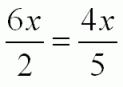SEARCH HOMEMath Central Quandaries & QueriesSubject: Algebra Name: B.Wilson Who are you: Parent The teacher has asked to solve the following, but I have never seen this before: 6x/2 = 4x/5Hi B.,

You have an equation to solve:One way to tackle this question is to first consider that the left side of the equation reduces to 3x.  So, you are looking for a number such that three times the number is the same as four fifths of the number. How can that be? If you triple a number, you should get a number greater than or equal to what you started with. If you take four fifths of a number, you should get a number that is less than or equal to the number you started with. So, what could x possibly be?

If you need a further hint, when using the above style of reasoning, it can be useful to think about separate cases for x: How would the equation work if x was positive? What if x was negative? What case am I leaving out?

HaleyMath Central is supported by the University of Regina and The Pacific Institute for the Mathematical Sciences.